# Pulley

On wheels with a diameter of 40 cm is fixed rope with the load. Calculate how far is load lifted when the wheel turns 7 times?

d =  8.8 m

### Step-by-step explanation: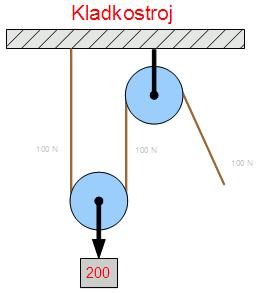We will be pleased if You send us any improvements to this math problem. Thank you!Tips to related online calculators
Do you want to convert length units?

#### You need to know the following knowledge to solve this word math problem:

We encourage you to watch this tutorial video on this math problem:

## Related math problems and questions:

• WellRope with a bucket is fixed on the shaft with the wheel. The shaft has a diameter 50 cm. How many meters will drop bucket when the wheels turn 15 times?
• A bicycle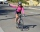A bicycle wheel has a diameter of 63 cm. Calculate how many times the wheel turns round in travelling 19.8 km .
• Bicycle wheel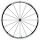Bicycle wheel diameter is 62 cm. How many times turns the bicycle on the road 1 km long?
• Winch drum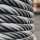Originally an empty winch drum with a diameter of 20 cm and a width of 30 cm on the rescue car, he started winding a rope with a thickness of 1 cm beautifully from edge to edge. The winch stopped after 80 turns. It remains to spin 3.54m of rope (without h
• Coal mine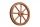The towing wheel has a diameter of 1.7 meters. How many meters does the elevator cage lower when the wheel turns 32 times?
• Wheel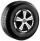What is the wheel diameter if on the 0.57 km track turns 106 times?
• Central angleWhat is the length of the arc of a circle with a diameter of 46 cm, which belongs to a central angle of 30°?
• Bike wheel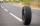The bike wheel has a radius of 30cm. How many times does it turn if we go on a 471m bike?
• Wheel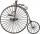Diameter of motocycle wheel is 52 cm. How many times rotates wheel on roand long 2 km?
• Diameter of a cylinderThe diameter of the cylinder is 42 cm. How many times does the cylinder turn on a 66m long track?
• Washer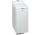The washing machine drum washes at 71 RPM. The washing machine motor pulley has a diameter of 8 cm. What must be the diameter of the drum machine pulley when the motor is at 351 RPM?
• TrianglesEquilateral triangle with side 40 cm has the same perimeter as an isosceles triangle with arm of 45 cm. Calculate the base x of an isosceles triangle.
• Bicycle wheelA bicycle wheel has a diameter of 60 cm. Approximately how many times does the wheel rotate at 2.5 km long trip?
• BaseBase of building is circle with diameter 25 m. Calculate the circumference of a circular trench witch diameter is 41 cm wider than the diameter of the base.
• Odometer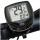The odometer is driven by the rotation of the wheel, whose diameter is 65 cm. After how many rotations, the wheel turns counter to the next kilometer?
• Wheel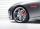How many times turns the wheel of a passenger car in one second if car run at speed 100 km/h. Wheel diameter is d = 62 cm.
• Bicycle wheelAfter driving 157 m bicycle wheel rotates 100 times. What is the radius of the wheel in cm?